Closure and interior

Closure and interior are two topological operators, defined over intuitionistic fuzzy sets, as follows.

Let$A \subset E$ be an IFS. Then,$C(A) = \lbrace \langle x, \sup_{y \in E} \mu_A(y), \inf_{y \in E} \nu_A(y) \rangle \ | \ x \in E \rbrace$$I(A) = \lbrace \langle x, \inf_{y \in E} \mu_A(y), \sup_{y \in E} \nu_A(y) \rangle \ | \ x \in E \rbrace$

are respectively called closure and interior.

The following basic statements are valid:

•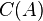$C(A)$ and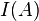$I(A)$ are intuitionistic fuzzy sets.
•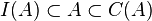$I(A) \subset A \subset C(A)$
•$C(C(A)) \ = \ C(A)$
•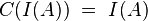$C(I(A)) \ = \ I(A)$
•$I(C(A)) \ = \ C(A)$
•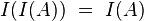$I(I(A)) \ = \ I(A)$

When operations and relations are applied over the closure and interior operators, the following valid statements can be formulated:

•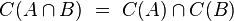$C(A \cap B) \ = \ C(A) \cap C(B)$
•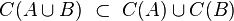$C(A \cup B) \ \subset \ C(A) \cup C(B)$
•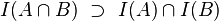$I(A \cap B) \ \supset \ I(A) \cap I(B)$
•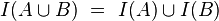$I(A \cup B) \ = \ I(A) \cup I(B)$
•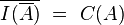$\overline{I(\overline{A})} \ = \ C(A)$

Further, when the modal operators necessity and possibility are applied, it holds that:

•$\Box (C(A)) \ = \ C(\Box(A))$
•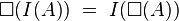$\Box (I(A)) \ = \ I(\Box(A))$
•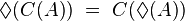$\Diamond (C(A)) \ = \ C(\Diamond (A))$
•$\Diamond (I(A)) \ = \ I(\Diamond (A))$

If$A$ and$B$ are intuitionistic fuzzy sets over$E$, the following statements hold about them:

• If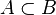$A \subset B$, then$I(A) \subset I(B)$ and$C(A) \subset C(B)$.